## Finite Difference

The finite difference is the discrete analog of the Derivative. The finite Forward Difference of a functionis defined as(1)

and the finite Backward Difference as(2)

If the values are tabulated at spacings, then the notation(3)

is used. Theth Forward Difference would then be written as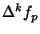, and similarly, theth Backward Difference as.

However, whenis viewed as a discretization of the continuous function, then the finite difference is sometimes written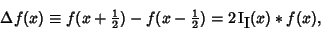(4)

wheredenotes Convolution andis the odd Impulse Pair. The finite difference operator can therefore be written(5)

Anth Power has a constantth finite difference. For example, takeand make a Difference Table,(6)

The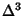column is the constant 6.

Finite difference formulas can be very useful for extrapolating a finite amount of data in an attempt to find the general term. Specifically, if a function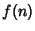is known at only a few discrete values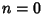, 1, 2, ... and it is desired to determine the analytical form of, the following procedure can be used ifis assumed to be a Polynomial function. Denote theth value in the Sequence of interest by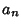. Then defineas the Forward Difference,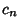as the second Forward Difference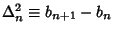, etc., constructing a table as follows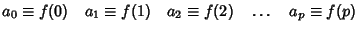(7)
Continue computing,, etc., until a 0 value is obtained. Then the Polynomial function giving the valuesis given by(8)

When the notation,, etc., is used, this beautiful equation is called Newton's Forward Difference Formula. To see a particular example, consider a Sequence with first few values of 1, 19, 143, 607, 1789, 4211, and 8539. The difference table is then given by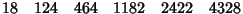Reading off the first number in each row gives,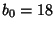,,,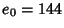. Plugging these in gives the equation(9)

which simplifies to, and indeed fits the original data exactly!

Beyer (1987) gives formulas for the derivatives(10)

(Beyer 1987, pp. 449-451) and integrals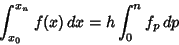(11)

(Beyer 1987, pp. 455-456) of finite differences.

Finite differences lead to Difference Equations, finite analogs of Differential Equations. In fact, Umbral Calculus displays many elegant analogs of well-known identities for continuous functions. Common finite difference schemes for Partial Differential Equations include the so-called Crank-Nicholson, Du Fort-Frankel, and Laasonen methods.

See also Backward Difference, Bessel's Finite Difference Formula, Difference Equation, Difference Table, Everett's Formula, Forward Difference, Gauss's Backward Formula, Gauss's Forward Formula, Interpolation, Jackson's Difference Fan, Newton's Backward Difference Formula, Newton-Cotes Formulas, Newton's Divided Difference Interpolation Formula, Newton's Forward Difference Formula, Quotient-Difference Table, Steffenson's Formula, Stirling's Finite Difference Formula, Umbral Calculus

References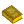Finite Difference Equations

Abramowitz, M. and Stegun, C. A. (Eds.). Differences.'' §25.1 in Handbook of Mathematical Functions with Formulas, Graphs, and Mathematical Tables, 9th printing. New York: Dover, pp. 877-878, 1972.

Beyer, W. H. CRC Standard Mathematical Tables, 28th ed. Boca Raton, FL: CRC Press, pp. 429-515, 1987.

Boole, G. and Moulton, J. F. A Treatise on the Calculus of Finite Differences, 2nd rev. ed. New York: Dover, 1960.

Conway, J. H. and Guy, R. K. Newton's Useful Little Formula.'' In The Book of Numbers. New York: Springer-Verlag, pp. 81-83, 1996.

Iyanaga, S. and Kawada, Y. (Eds.). Interpolation.'' Appendix A, Table 21 in Encyclopedic Dictionary of Mathematics. Cambridge, MA: MIT Press, pp. 1482-1483, 1980.

Jordan, K. Calculus of Finite Differences, 2nd ed. New York: Chelsea, 1950.

Levy, H. and Lessman, F. Finite Difference Equations. New York: Dover, 1992.

Milne-Thomson, L. M. The Calculus of Finite Differences. London: Macmillan, 1951.

Richardson, C. H. An Introduction to the Calculus of Finite Differences. New York: Van Nostrand, 1954.

Spiegel, M. Calculus of Finite Differences and Differential Equations. New York: McGraw-Hill, 1971.

© 1996-9 Eric W. Weisstein
1999-05-26